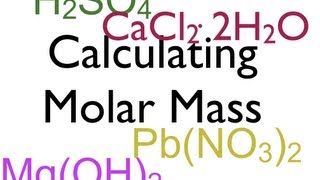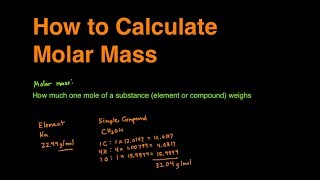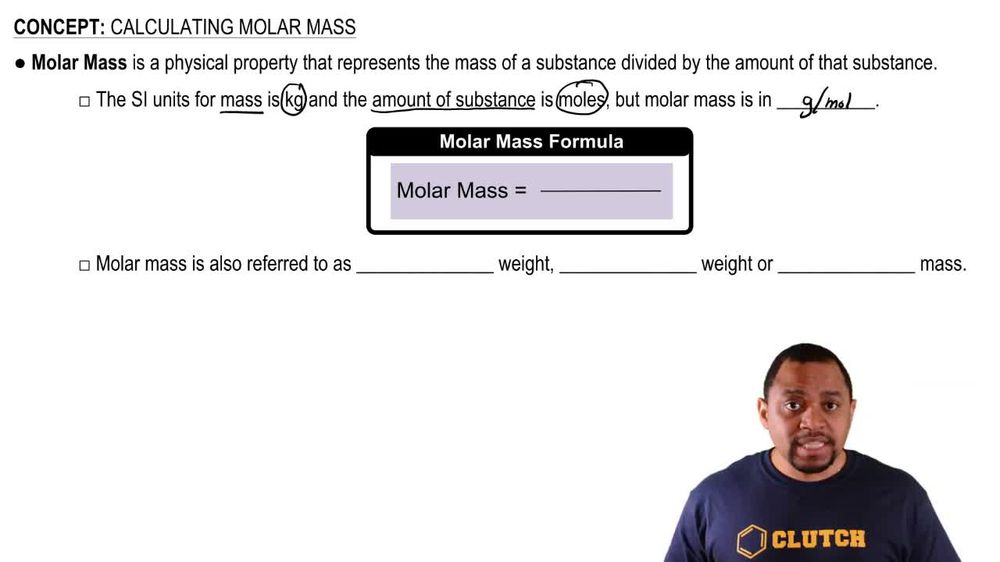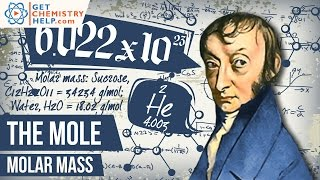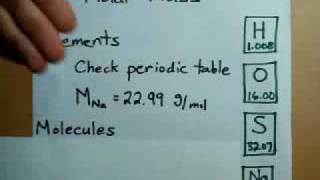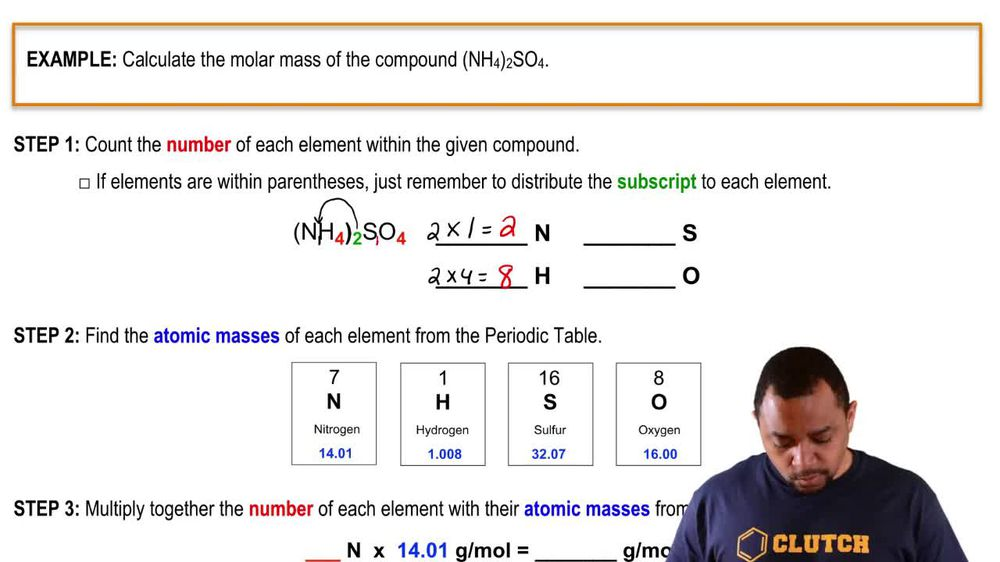Start typing, then use the up and down arrows to select an option from the list.
1. 2. Atoms & Elements2. Calculating Molar Mass
Problem

# What are the molecular weights of the following herbicides? (a) C6H6Cl2O3 (2, 4-dichlorophenoxyacetic acid, effective on broadleaf plants)

Relevant Solution2m
Play a video:
Welcome back everyone in this example, we're told that caffeine, a natural stimulant found in coffee and tea, has the following molecular formula and we need to calculate the molar mass of caffeine. So we first want to recall that molar mass is represented by this symbol here, A big capital M. And we can calculate this only in units of grams per mole. We should recall because it's for one mole of our substance. So we can find this by taking our number of atoms of our element, which is given in our molecular formula in the prompt. And we would multiply this by their respective atomic mass which we should recall. We can find on our periodic table and so going into our molecular formula, we see that we have first eight atoms of carbon. So we're going to multiply this by its respective molar mass on the periodic table given as 12.01 g per mole. Now because our molecular formula consists of not just carbon. We're going to place this in parentheses and add onto this. Our next atom, which we can see is our 10 atoms of hydrogen. So we have 10 atoms of hydrogen. We're going to multiply it by their respective Mueller mass on the periodic table given as one point oh eight g per mole. Moving forward, we have our next atom where we see, we have four atoms of nitrogen. So we can see added onto this will say four atoms of nitrogen multiplied by the respective molar mass on the periodic table given as 14.01 g per mole finishing off our parentheses here, we just have one last element in our molecular formula where we see we have two atoms of oxygen. So we have added onto this two Atoms of oxygen And this is going to be multiplied by its respective molar mass given on the periodic table as a value of 16. And we're going to use units of g per mole. And so to complete our calculation, we're going to type in Our entire expression above and this is going to yield a result equal to a value of 192, correction 194 0.2, and we have units of g per mole. And so this would be our final answer for our calculation of our molar mass of caffeine. So I hope that everything that I reviewed was clear. If you have any questions, please leave them down below and I will see everyone in the next practice video.